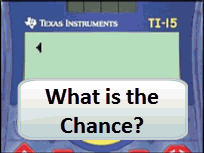# Activities

•• ##### Subject Area

• Standard: National Curriculum 7-10: Statistics and Probability: Chance

• ##### AuthorPrimary

60 Minutes

• ##### Device
• TI-15 Explorer™

## What's the Chance#### Activity Overview

Students use spinners, dice and cards to simulate probability events. A sample space is collected the probabilities estimated using both fractions and percentage.

#### Objectives

Assign probabilities to the outcomes of events and determine probabilities for events - ACMSP168

#### Vocabulary

Likely, unlikely, impossible, certain, expected,

#### About the Lesson

Students experiment with cards, spinners and dice to generate a sample space for each event. The outcomes are used to estimate the probability as a fraction and then converted to a percentage.
Documents include Teacher notes, curriculum content, worksheets, assessment tasks and solutions